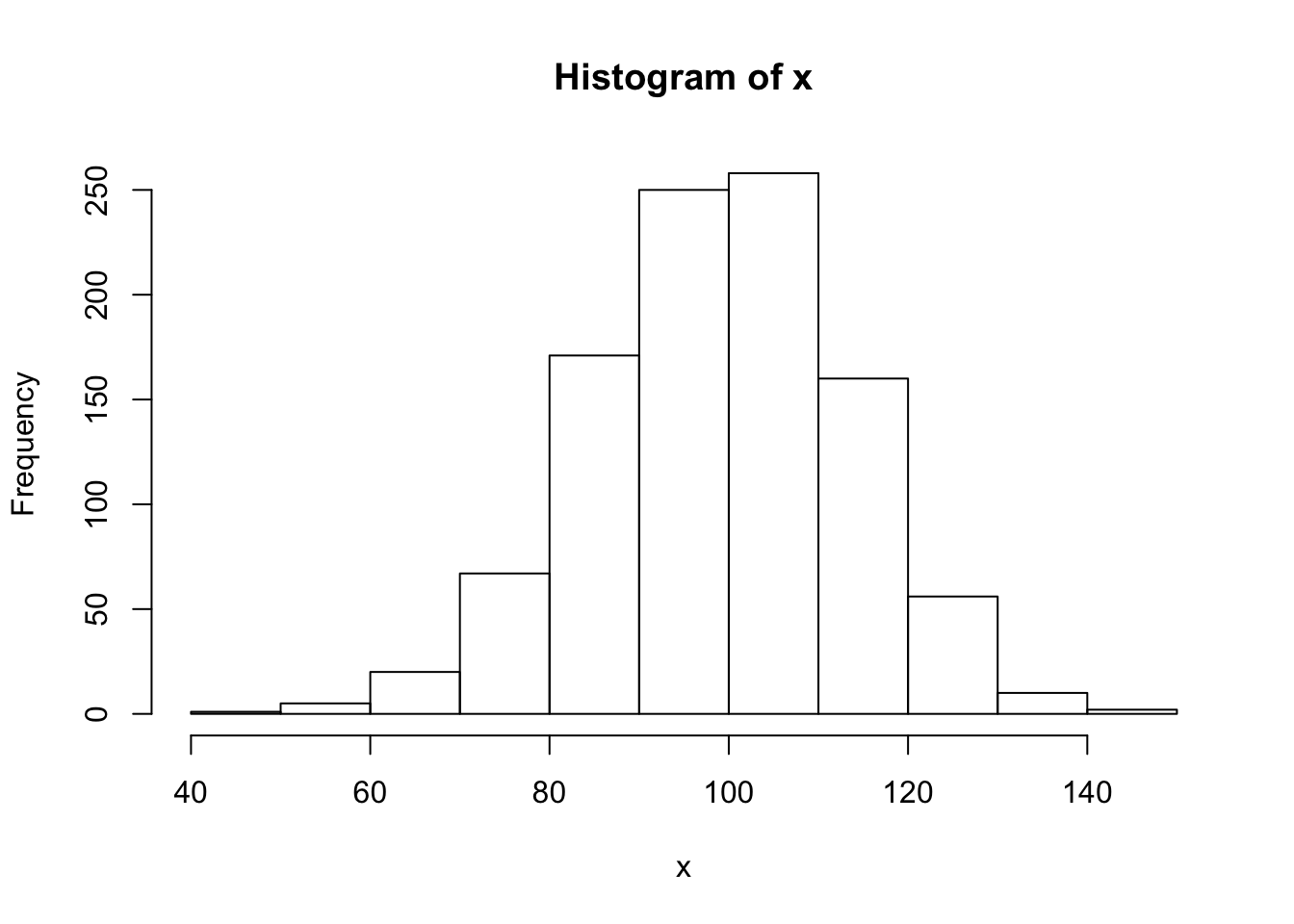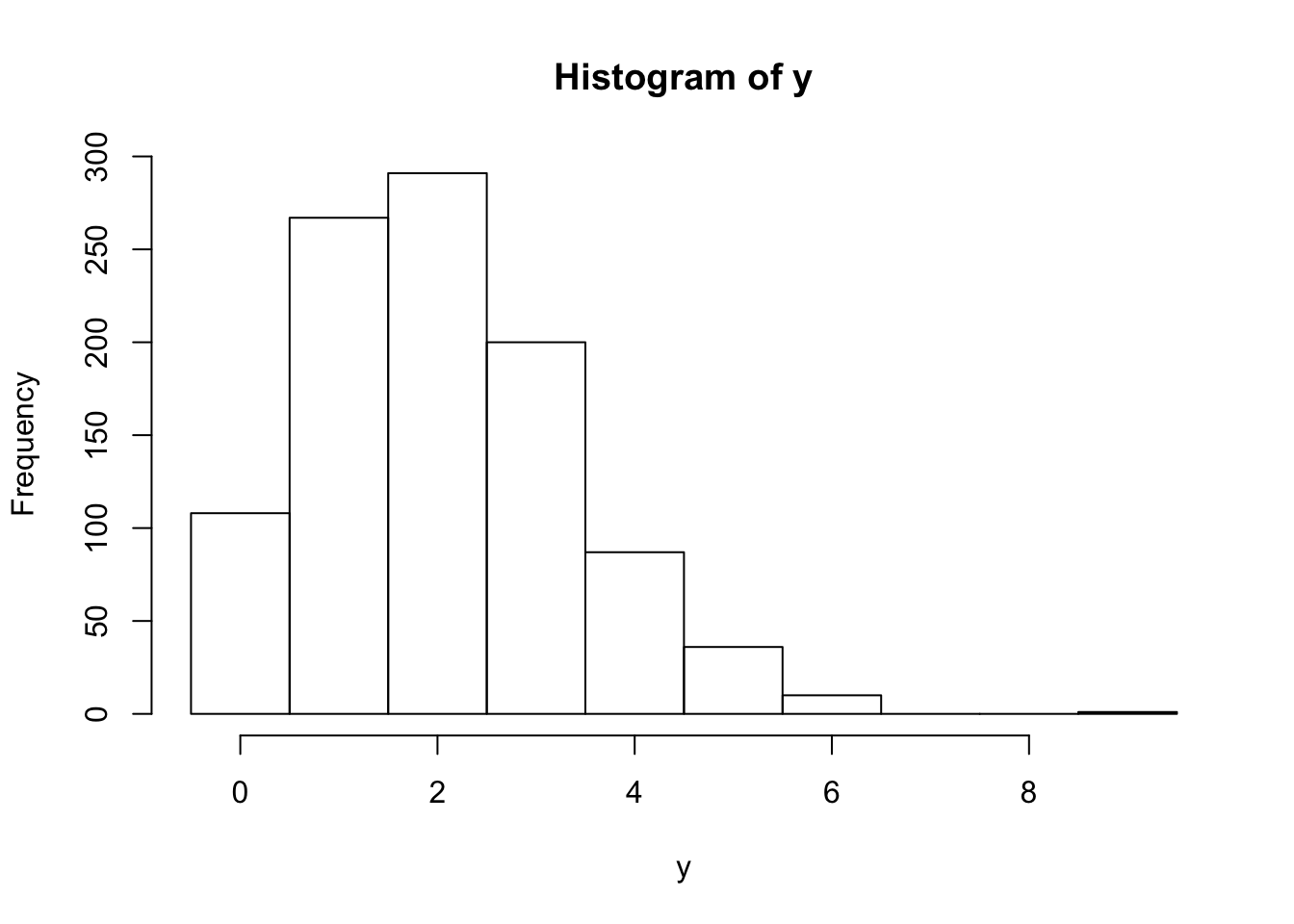The rand_bytes function binds to RAND_bytes in OpenSSL to generate cryptographically strong pseudo-random bytes. See the OpenSSL documentation for what this means.

rnd <- rand_bytes(10)
print(rnd)
  25 6f fc 3f 2c a7 36 3e 30 ab

Bytes are 8 bit and hence can have 2^8 = 256 possible values.

as.numeric(rnd)
   37 111 252  63  44 167  54  62  48 171

Each random byte can be decomposed into 8 random bits (booleans)

x <- rand_bytes(1)
as.logical(rawToBits(x))
 FALSE  TRUE  TRUE  TRUE FALSE  TRUE FALSE FALSE

## Secure Random Numbers

rand_num is a simple (2 lines) wrapper to rand_bytes to generate random numbers (doubles) between 0 and 1.

rand_num(10)
  0.7709538 0.6803416 0.2199021 0.5627981 0.8837980 0.4052726 0.1712342
 0.7817140 0.1486292 0.8552378

To map random draws from [0,1] into a probability density, we can use a Cumulative Distribution Function. For example we can combine qnorm and rand_num to simulate rnorm:

# Secure rnorm
x <- qnorm(rand_num(1000), mean = 100, sd = 15)
hist(x)Same for discrete distributions:

# Secure rbinom
y <- qbinom(rand_num(1000), size = 20, prob = 0.1)
hist(y, breaks = -.5:(max(y)+1))